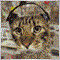• 概要
• レビュー (1)
• コメント
• 最新情報

Universal Advisor. Works as a scalper and dynamic order grid. Applies levels of stop orders, stop loss, take profit and trailing stop that are invisible to the broker. Level values can be given in pips or percent of average price volatility (ATR). It can work at a specified time, has protection against slipping and expansion of the spread. The graph displays information about the quality of execution of orders, profits and current open positions (the number of orders, the number of lots and profit). Suitable for working with any brokers, including American brokers with the requirement of FIFO.

### Money Management:

• FIX_START_LOT - fixed starting lot for each N amount from the balance (FROM_BALANCE);
• FROM_BALANCE - N balance amount for the calculation of the starting lot (FIX_START_LOT);

The starting lot is calculated by the formula: Balance / FROM_BALANCE * FIX_START_LOT

Example number 1:

• Balance 300
• FIX_START_LOT - 0.01;
• FROM_BALANCE - 100;

Starting lot will be = 0.03.

Example number 2:

• Balance 300
• FIX_START_LOT - 0.01;
• FROM_BALANCE - 300;

Starting lot will be = 0.01.

### Dynamic order grid:

• LOT_MULTIPLY - lot multiplier in the order grid;
• START_MULTIPLY - from which order to start increasing the lot;
• MIN_GRID_STEP - the minimum step between orders;
• GRID_PRICE_STEP - the minimum step for the price movement;

Example number 1:

• MIN_GRID_STEP = 100;
• GRID_PRICE_STEP = 200;

When the price passes 300 (MIN_GRID_STEP + GRID_PRICE_STEP) pips in the opposite direction from the last open order, the virtual level of the STOP order will be established - the price of the last open order +/- MIN_GRID_STEP. The order will open at the set level, but if the price continues to move in the opposite direction by N pips (MIN_GRID_STEP + GRID_PRICE_STEP), the level of the STOP order will change - the price of the last level +/- MIN_GRID_STEP pips.

Example number 2:

• LOT_MULTIPLY = 1.5;
• START_MULTIPLY = 3;

The order lot will start to increase from the N order (START_MULTIPLY). The 1st order with lot 0.1 is opened, the 2nd order will also be opened with lot 0.1, and the 3rd order already with lot 0.15. And starting with N orders (START_MULTIPLY), the lot will increase by LOT_MULTIPLY. The last lot multiplied by START_MULTIPLY.

### Opening the first order:

• DIST_TO_OPEN - distance from the Ask / Bid price;
• TIMER_SECONDS - timer to modify levels;

The Expert Advisor sets the levels at N distance (DIST_TO_OPEN) from the Ask / Bid price, which, when touched, opens the first trade. Levels are modified by timer TIMER_SECONDS. If for the allotted time the price failed to break through the specified level, then they are modified at the new prices.

### Other parameters:

• ATR_VALUES - use the percentage of ATR to calculate levels;
• PERIOD_ATR - ATR period;
• MAGIC_NUMBER - magic number;
• TIME_START , TIME_END - the start and end time of the advisor, at 00:00 is not used;
• MAX_SLIPPAGE - maximum slippage for the first order, in pips. when = 0, not used;
• MAX_SPREAD - the maximum size of the spread when opening orders, in pips;
• STOPLOSS - stop loss of the order grid, in pips or% of ATR if ATR_VALUES = true. when = 0, not used;
• TAKEPROFIT - take profit of the order grid, in pips or% of ATR if ATR_VALUES = true. when = 0, not used;
• TRAILING_STOP - minimum profit during trailing, in pips or% from ATR if ATR_VALUES = true. when = 0, not used;
• TRAILING_STEP - trailing step, in pips or% of ATR if ATR_VALUES = true;

おすすめの製品
フィルタ:629
2019.02.15 13:00

ユーザーはレーティングに対して何もコメントを残しませんでした

バージョン 1.1 2019.02.03
Исправлена небольшая опечатка в коде